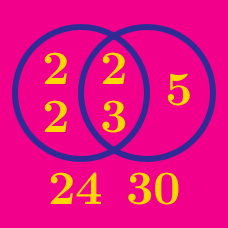Number Theory

# Greatest Common Divisor / Lowest Common Multiple: Level 3 Challenges

If $$x$$ and $$y$$ are integers, what is the smallest possible positive value of $$30x+18y?$$

$\huge \color{red}{2}, \color{green}{-4}$

Find the lowest common multiple of the two numbers above.

$\LARGE \color{blue}{0.\bar{3}}, \color{red}{0.\bar{5}}$

What is the lowest common multiple of the two numbers given above?

Note that $$0. \overline{ABC} = 0.ABCABCABC\ldots$$.

How many positive integers $$a$$ are there such that 2027 divided by $$a$$ leaves a remainder of 7?

Evaluate $$\gcd ( 19 ! + 19, 20! + 19 )$$.

Details and assumptions

The number $$n!$$, read as n factorial, is equal to the product of all positive integers less than or equal to $$n$$. For example, $$7! = 7 \times 6 \times 5 \times 4 \times 3 \times 2 \times 1$$.

How many ordered pairs of positive integers $$(m, n)$$ satisfy

$\gcd (m^3, n^2) = 2^2 \cdot 3^2 \text{ and } \text{lcm}(m^2, n^3) = 2^4 \cdot 3^4 \cdot 5^6?$

This problem is shared by Muhammad A.

Details and assumptions

$$\gcd(a, b)$$ and $$\text{lcm}(a, b)$$ denote the greatest common divisor and least common multiple of $$a$$ and $$b$$, respectively.

×